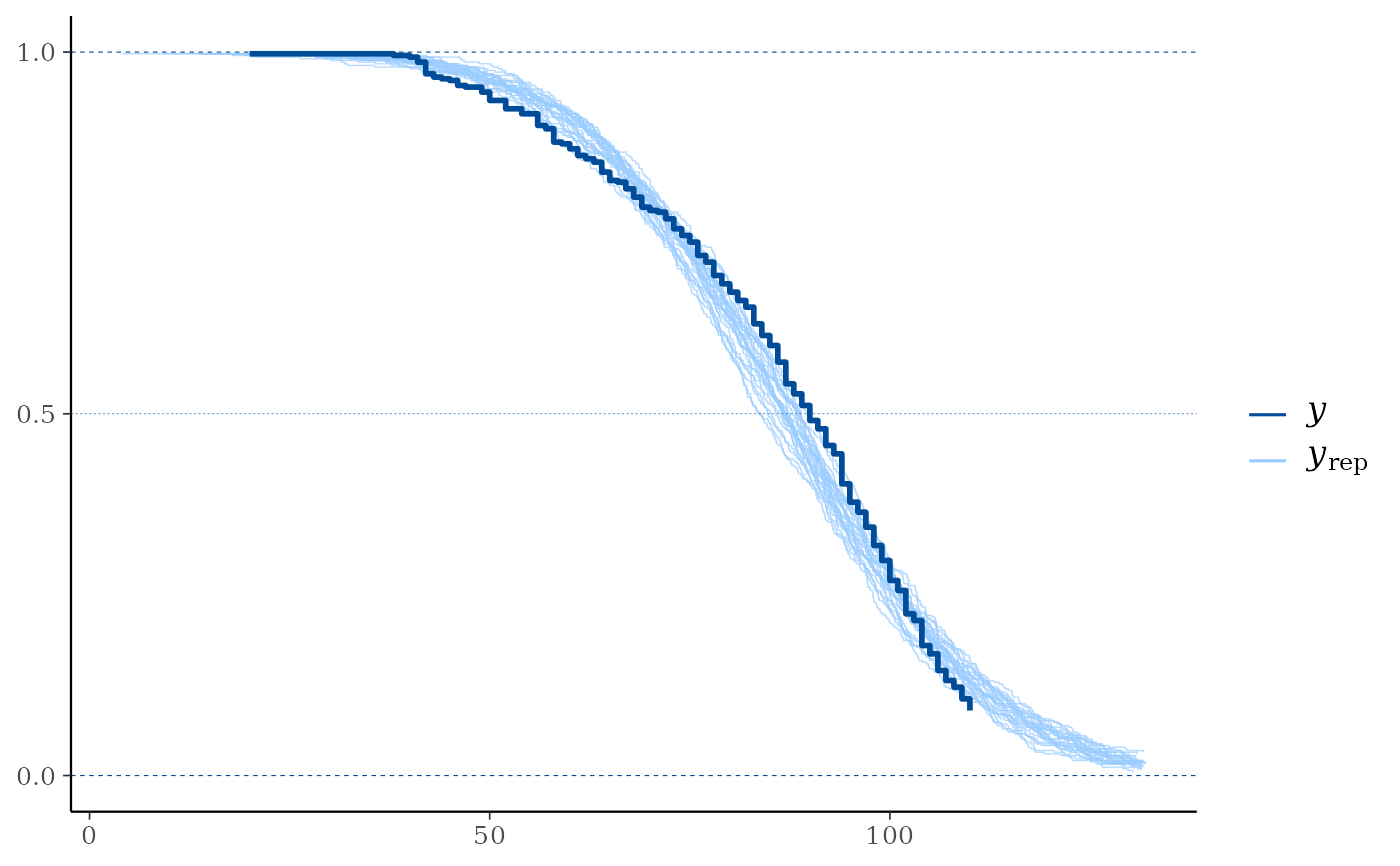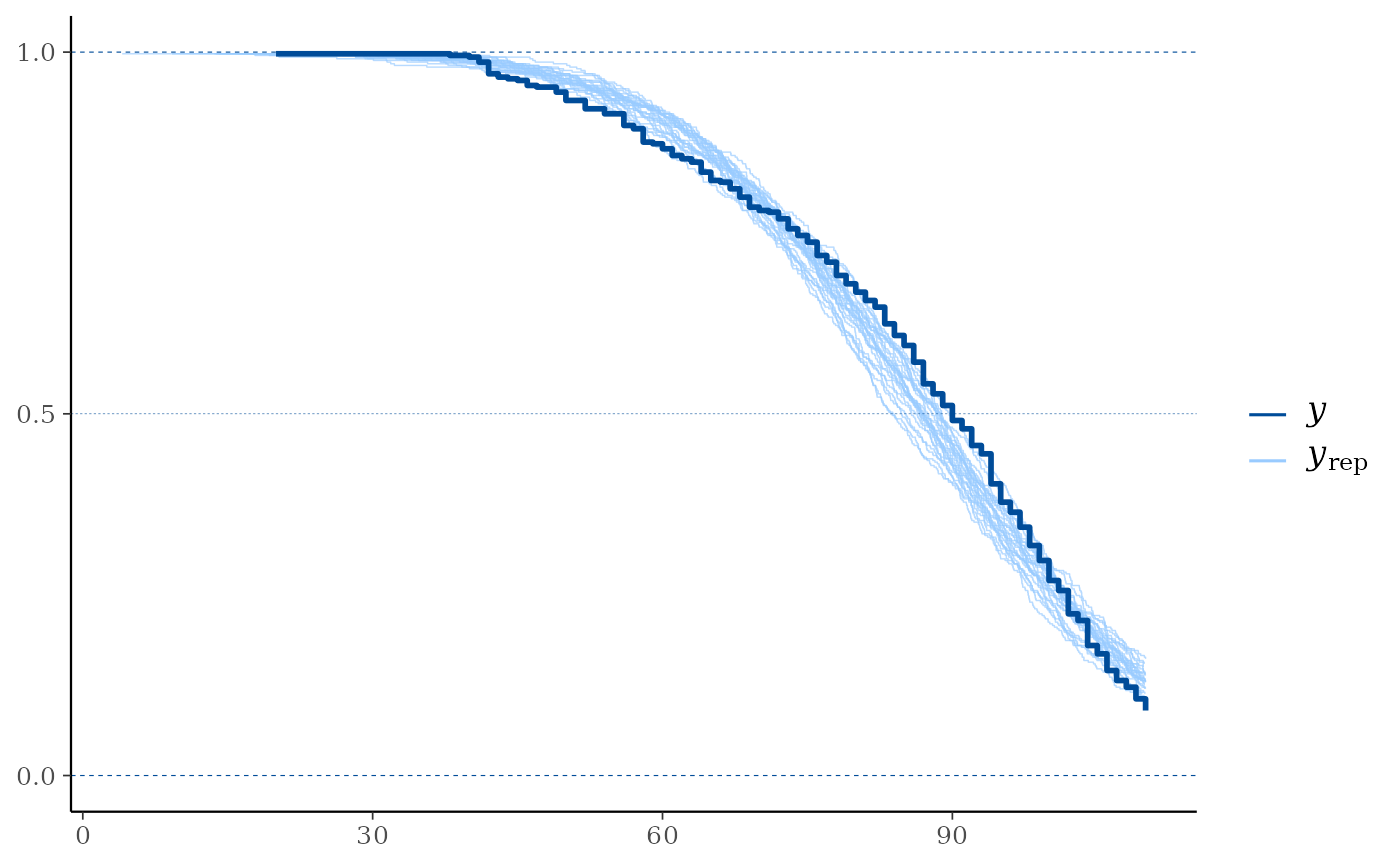Compare the empirical distribution of censored data y to the distributions of simulated/replicated data yrep from the posterior predictive distribution. See the Plot Descriptions section, below, for details.

Although some of the other bayesplot plots can be used with censored data, ppc_km_overlay() is currently the only plotting function designed specifically for censored data. We encourage you to suggest or contribute additional plots at github.com/stan-dev/bayesplot.

ppc_km_overlay(y, yrep, ..., status_y, size = 0.25, alpha = 0.7)

ppc_km_overlay_grouped(y, yrep, group, ..., status_y, size = 0.25, alpha = 0.7)

## Arguments

y

A vector of observations. See Details.

yrep

An S by N matrix of draws from the posterior (or prior) predictive distribution. The number of rows, S, is the size of the posterior (or prior) sample used to generate yrep. The number of columns, N is the number of predicted observations (length(y)). The columns of yrep should be in the same order as the data points in y for the plots to make sense. See the Details and Plot Descriptions sections for additional advice specific to particular plots.

...

Currently only used internally.

status_y

The status indicator for the observations from y. This must be a numeric vector of the same length as y with values in {0, 1} (0 = right censored, 1 = event).

size, alpha

Passed to the appropriate geom to control the appearance of the yrep distributions.

group

A grouping variable of the same length as y. Will be coerced to factor if not already a factor. Each value in group is interpreted as the group level pertaining to the corresponding observation.

## Value

A ggplot object that can be further customized using the ggplot2 package.

## Plot Descriptions

ppc_km_overlay()

Empirical CCDF estimates of each dataset (row) in yrep are overlaid, with the Kaplan-Meier estimate (Kaplan and Meier, 1958) for y itself on top (and in a darker shade). This is a PPC suitable for right-censored y. Note that the replicated data from yrep is assumed to be uncensored.

ppc_km_overlay_grouped()

The same as ppc_km_overlay(), but with separate facets by group.

Other PPCs: PPC-discrete, PPC-distributions, PPC-errors, PPC-intervals, PPC-loo, PPC-overview, PPC-scatterplots, PPC-test-statistics

## Examples

color_scheme_set("brightblue")
y <- example_y_data()
# For illustrative purposes, (right-)censor values y > 110:
status_y <- as.numeric(y <= 110)
y <- pmin(y, 110)
# In reality, the replicated data (yrep) would be obtained from a
# model which takes the censoring of y properly into account. Here,
# for illustrative purposes, we simply use example_yrep_draws():
yrep <- example_yrep_draws()
dim(yrep)
#>  500 434
# \donttest{
ppc_km_overlay(y, yrep[1:25, ], status_y = status_y)# }
# With separate facets by group:
group <- example_group_data()
# \donttest{
ppc_km_overlay_grouped(y, yrep[1:25, ], group = group, status_y = status_y)# }